# Problems of the Week

Contribute a problem

# 2017-07-10 Intermediate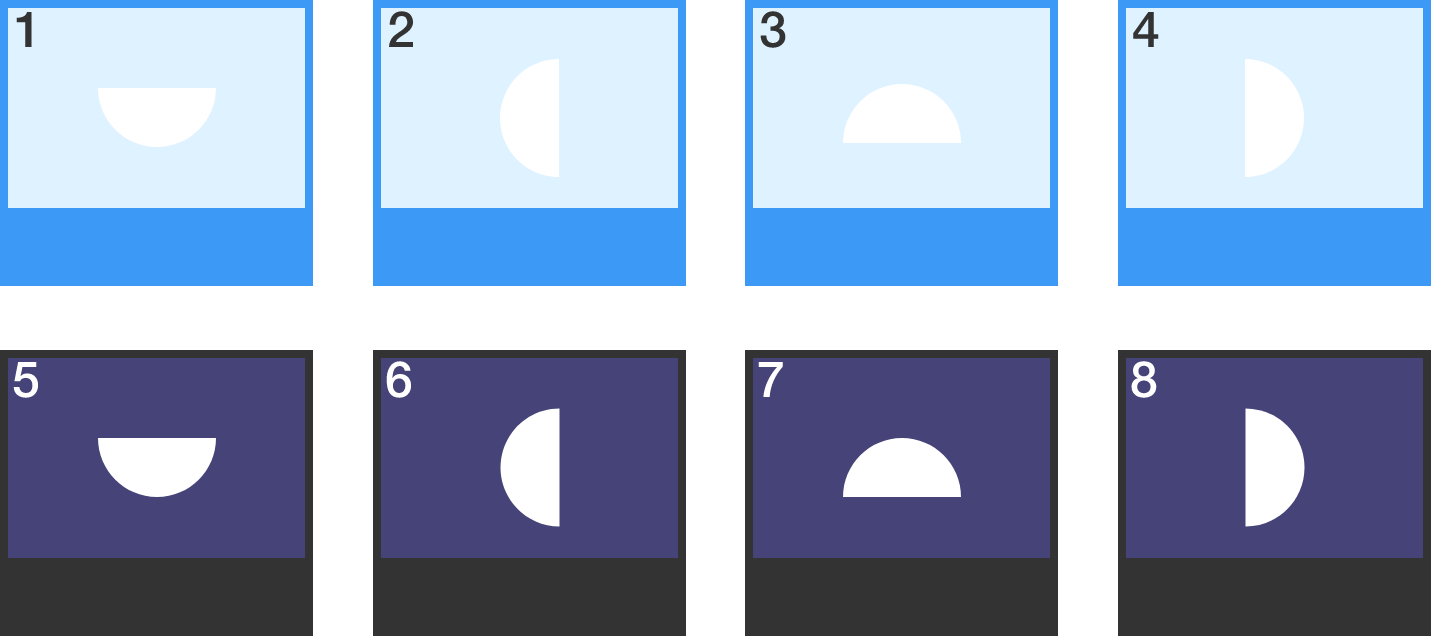Which of the images are possible views of a moon near the horizon (either rising or setting) close to the equator?

The top four images represent daytime, and the bottom four nighttime. The phase of the moon is not necessarily consistent across the images.

Suppose someone selects three points at random from the surface of a sphere. Then what is the the probability, in percent, that you can draw a great circle--the largest circle you can possibly draw on the sphere--such that all 3 of the points lie on the same hemisphere (divided by the great circle)?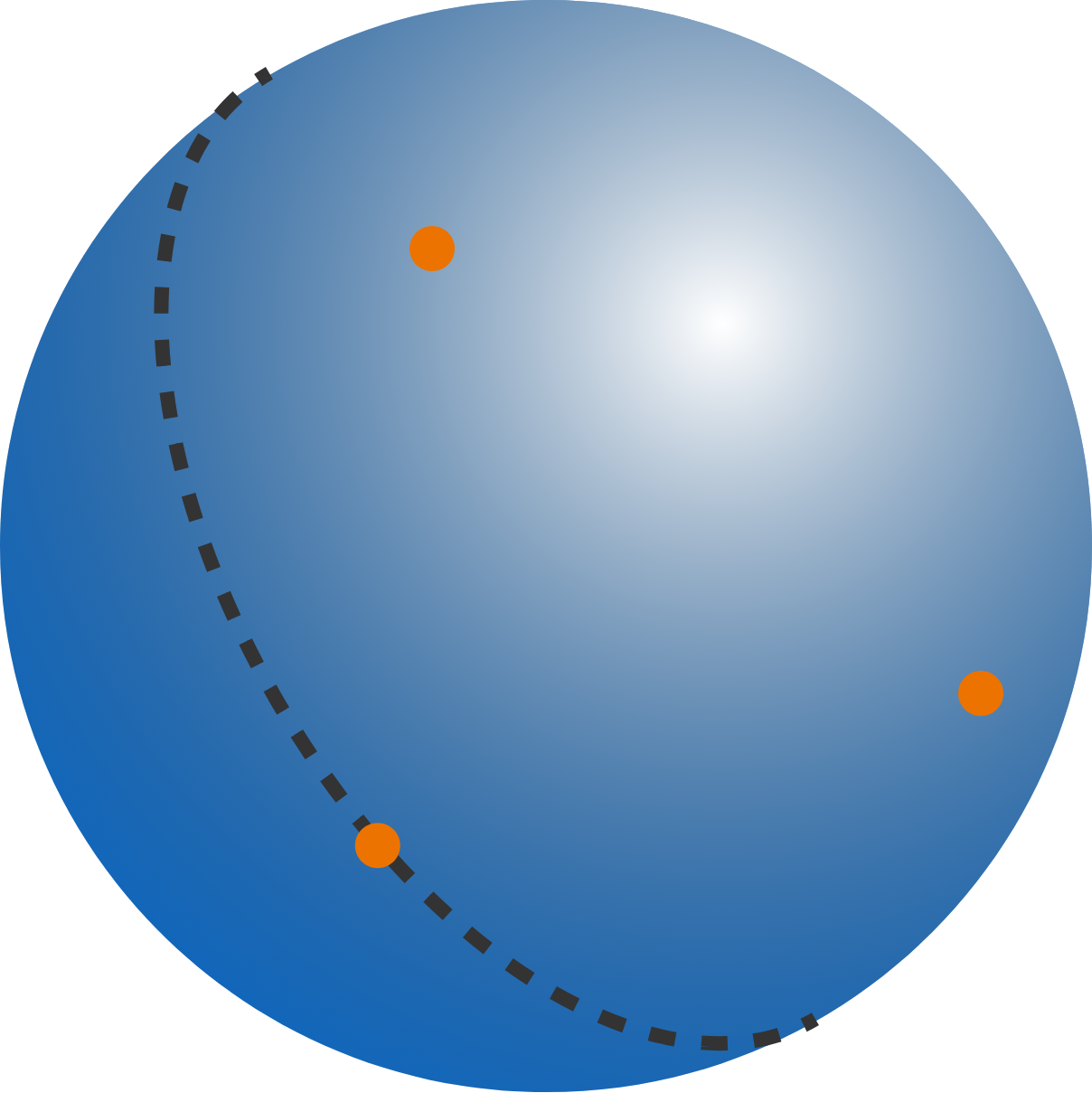Details and Assumptions:

• Points on the boundary of a hemisphere are considered to lie on the hemisphere.

• Enter, for example, percent like 50% as 50.

Is it possible for a Knight on a standard chess board to start at square H1 and touch every other square of the board exactly once (using standard Knight moves), finishing at square A8?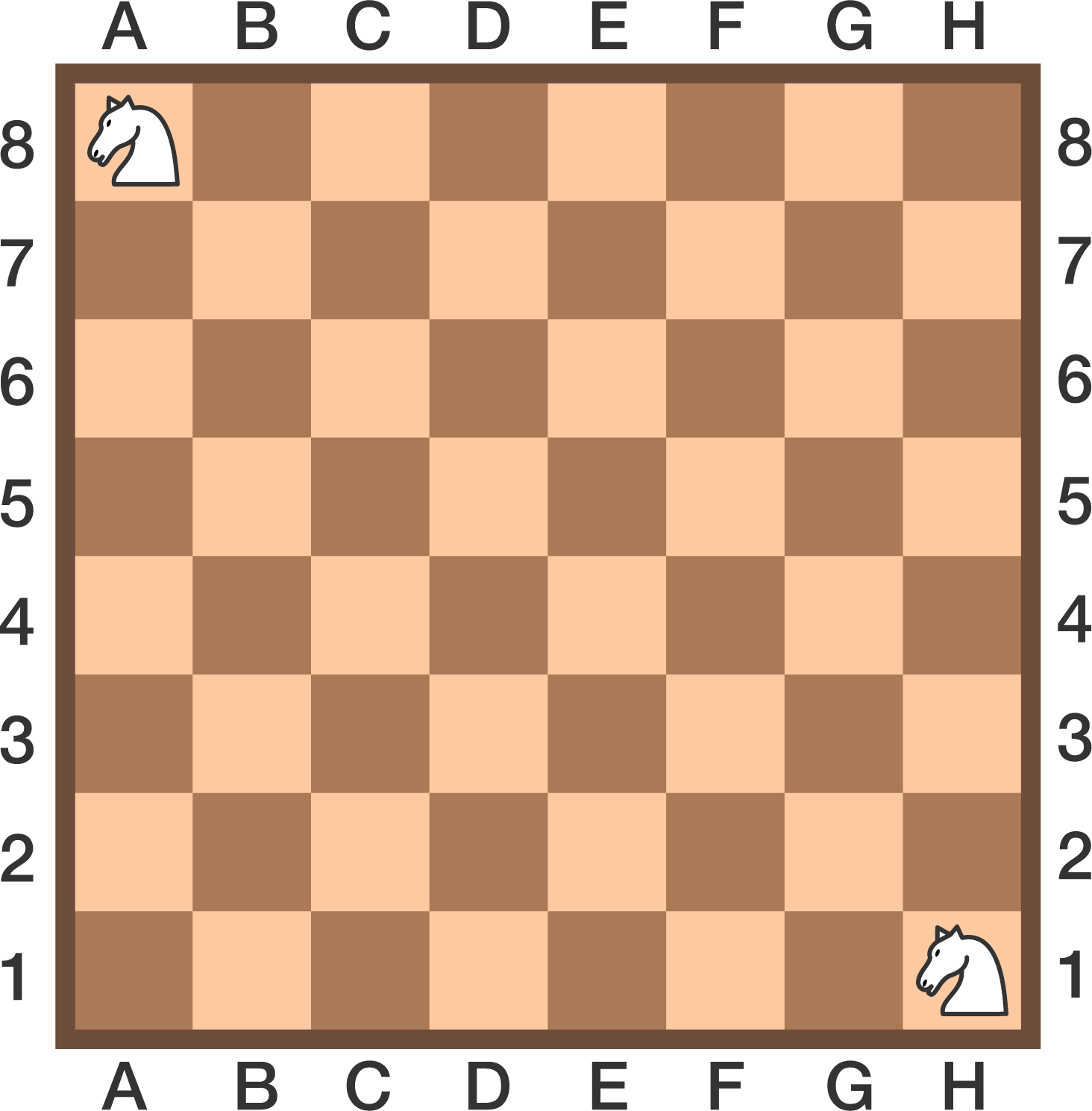4 circles overlap and divide one another into a total of 10 parts, as indicated by the 10 question marks in the diagram. Replace each question mark with a distinct digit 0 to 9, such that the sum of the digits in each circle is equal to the same value $x$.

Find the maximum possible value of $x$.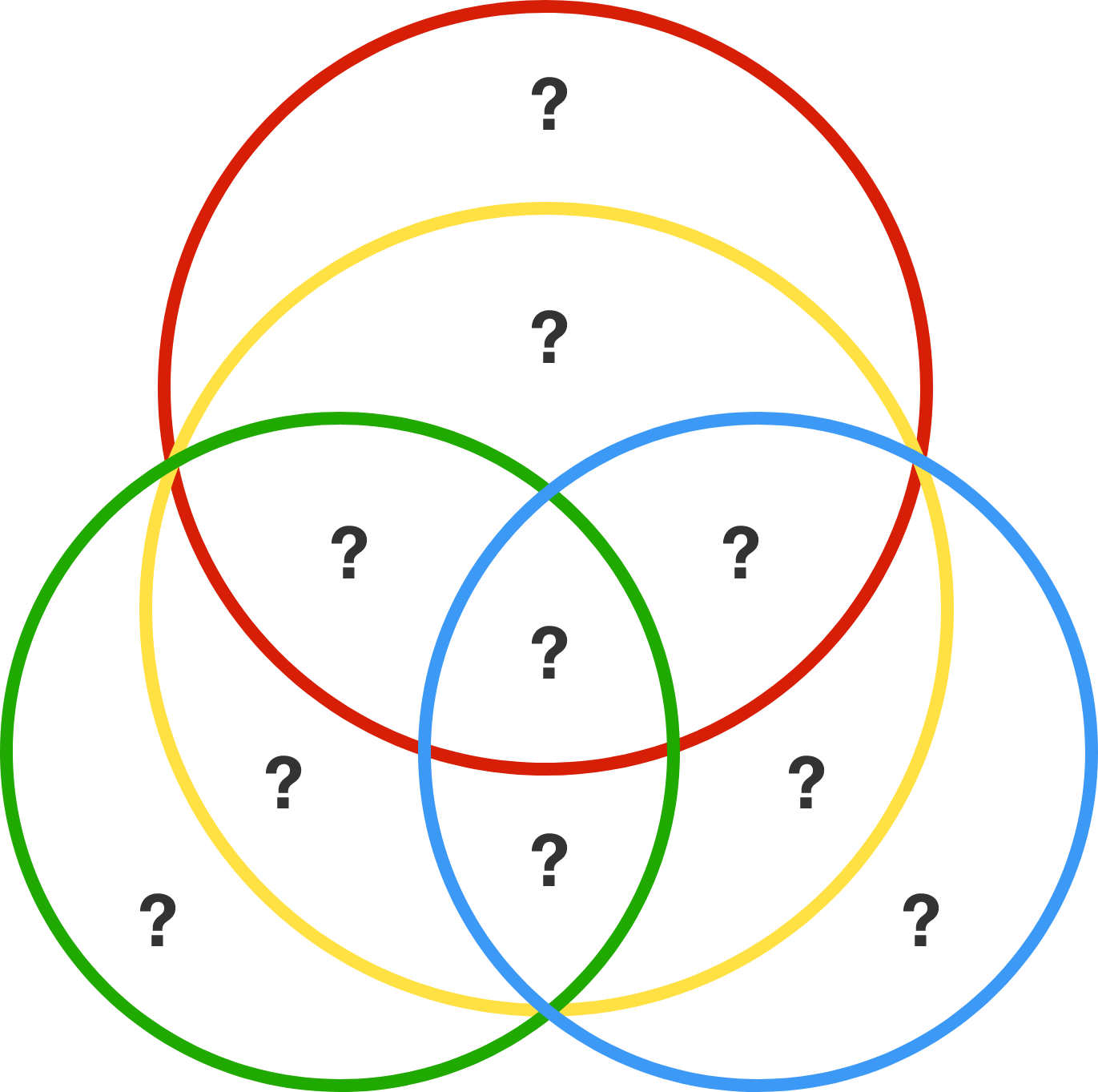Suppose planet BigEarth has the same density and distribution of mass as Earth, and that its atmosphere is identical to Earth's in composition and temperature.

If the radius of BigEarth is twice that of Earth, and the atmospheric pressure at its surface is $\SI{1}{atm},$ find the height of its atmosphere relative to that of Earth.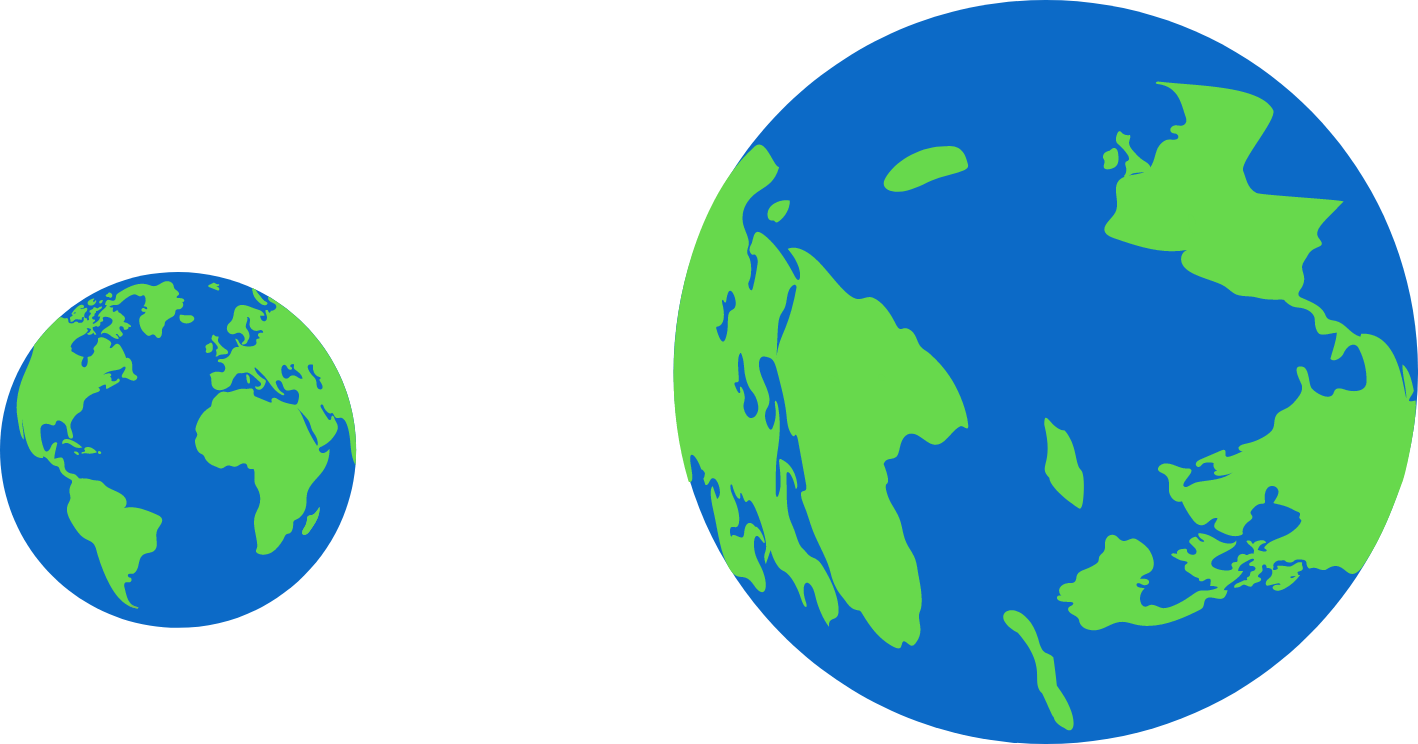×